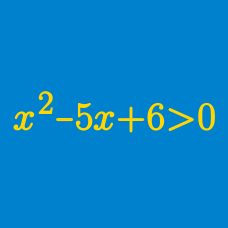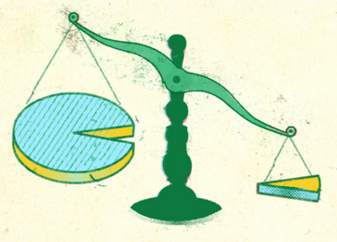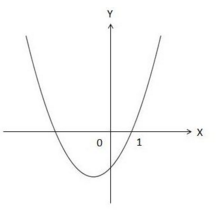Algebra

Polynomial Inequalities: Level 2 Challenges

Let $x$ be a real number such that the square root of $x$ is greater than the square of $x$. Which of the following is a possible value of $x$?

Find the interval of $v$ which satisfies the inequality $(v+2)^2(v-4)(v-6)<0.$

Consider the polynomial $p(x) = ax^2 + bx + c$ has no real roots. Provided that $25a+5b+c>0$, which of the following condition must be true?How many integer values of $u$ satisfy $u^4+u^3-12u^2<0?$The graph above is a quadratic function, $y =x^2+kx-2$ for some constant $k$. What is the solution to the quadratic inequality $x^2 + kx-2 < 0$?

Clarification: The graph intersects the $x$-axis at $x=1$.

×